# 4.2. 距离多普勒成像¶

## 4.2.1. 距离多普勒方法¶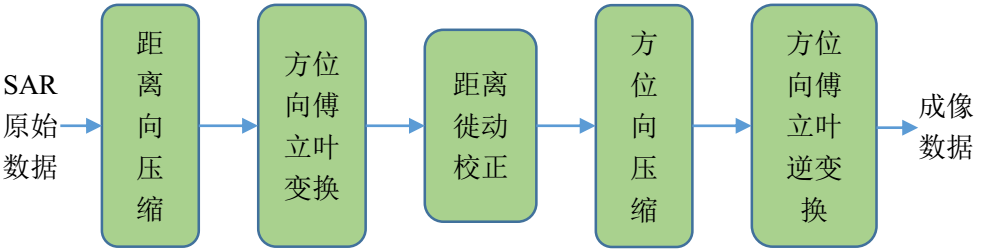### 小斜视下的RDA¶

#### SAR原始数据¶

SAR 原始数据是指SAR系统接收到的数据, 数据先被解调至基带, 距离频率中心被置零, 解调后的基带信号为 (参见: Section-SARSignalSystemOverviewSARRadar 小结, 式.2.35 )

\begin{aligned} s_i(\eta, \tau) &= G_i h(\tau, \eta)\\ &= G_i w_r\left(\tau-2R(\eta)/c\right) w_a(\eta-\eta_c)\\ & {\rm exp}\left\{ -j4\pi f_0 R(\eta)/c + j\pi K_r\left(\tau-2R(\eta)/c\right)^2 \right\}. \end{aligned}

#### 距离压缩¶

• 将SAR基带信号的距离维FFT变换 $$S_r(\eta, f_\tau)$$ 与距离向匹配滤波器 $$H_r(f_{\tau})$$ 相乘;

• 进行距离维逆FFT完成距离维压缩.

SAR回波信号 $$s(\eta, \tau)$$ 的距离维傅里叶变换为

\begin{aligned} S_r(\eta, f_{\tau}) &={\rm FFT}_{\tau}\left\{s(\eta, \tau)\right\}\\ &= G W_r(f_\tau)w_a(\eta-\eta_c) \\ & {\rm exp}\left\{-j\frac{4\pi(f_0+f_\tau)R(\eta)}{c}\right\}{\rm exp}\left\{-j\frac{\pi f_\tau^2}{K_r}\right\}, \end{aligned}

(4.2)$H_r(f_\tau) = {\rm exp}\left\{j\frac{\pi f_\tau^2}{K_r}\right\},$

(4.3)\begin{aligned} s_{rc}(\eta, \tau) &= {\rm IFFT}_{\tau}\left\{S_r(\eta, f_{\tau})H_r(f_{\tau})\right\} \\ &= G p_r\left(\tau-\frac{2R(\eta)}{c}\right)w_a(\eta-\eta_c) \\ & {\rm exp}\left(-j\frac{4\pi f_0 R(\eta)}{c}\right), \end{aligned}

#### 方位向傅里叶变换¶

$R(\eta) = \sqrt{R_0^2 +V_r^2\eta^2} \thickapprox R_0 + \frac{V_r^2\eta^2}{2R_0},$

$s_{rc}(\eta,\tau) \thickapprox G p_r(\tau-\frac{2R(\eta)}{c})w_a(\eta-\eta_c) \\ \qquad {\rm exp}\left(-j\frac{4\pi f_0 R_0}{c}\right) {\rm exp}\left(-j \frac{\pi 2V_r^2 \eta^2}{\lambda R_0}\right).$

\begin{aligned} S_a(f_{\eta}, \tau) &= {\rm FFT}_{\eta}\left\{s_{rc}(\eta, \tau)\right\} \\ &= G p_r(\tau-\frac{2R_{rd}(f_\eta)}{c})W_a(f_\eta-f_{\eta_c}) \\ & {\rm exp}\left(-j \frac{4\pi f_0 R_0}{c}\right) {\rm exp}\left(j \frac{\pi {f_\eta}^2}{K_a}\right), \end{aligned}

$R_{rd}(f_\eta) \thickapprox R_0 + \frac{V_r^2}{2R_0}\left(\frac{f_\eta}{K_a}\right)^2 = R_0 + \frac{\lambda^2R_0 f_{\eta}^2}{8V_r^2}.$

#### 距离徙动校正¶

$\Delta R(f_\eta) = \frac{\lambda^2R_0f_\eta^2}{8V_r^2}.$

(4.4)$D(f_{\eta}, V_r) = \sqrt{1-\frac{c^2f_{\eta}^2}{4V_r^2f_0^2}} = \sqrt{1- \frac{\lambda^2f_{\eta}^2 }{4V_r^2}}$

$Q_{rcmc}(f_\tau) = {\rm exp}\left(j\frac{4\pi f_\tau \Delta R(f_\eta)}{c}\right),$

\begin{aligned} S_{rcmc}(f_{\eta}, \tau) &= {\rm FFT}_{\eta}\left\{s_{rc}(\eta, \tau)\right\} \\ &= G p_r \left(\tau-\frac{2R_0}{c}\right)W_a(f_\eta-f_{\eta_c}) \\ & {\rm exp}\left(-j\frac{4\pi f_0 R_0}{c}\right) {\rm exp}\left(j\frac{\pi {f_\eta}^2}{K_a}\right). \end{aligned}

#### 方位压缩¶

• 将方位向FFT后的 $$S_a(f_{\eta}, \tau)$$ (或RCMC后的 $$S_{rcmc}(f_{\eta}, \tau)$$ ) 与方位向匹配滤波器 $$H_a(f_{\eta})$$ 相乘;

• 进行方位维逆FFT完成方位维压缩.

$H_{a}(f_\eta) = {\rm exp}\left\{-j\frac{\pi f_\eta^2}{K_a}\right\}.$

(4.5)\begin{aligned} {S_{af}}({f_\eta },\tau ) &= {S_{rcmc}}({f_\eta },\tau ){H_a}({f_\eta })\\ &= G{p_r}\left( {\tau - \frac{{2{R_0}}}{c}} \right){W_a}({f_\eta } - {f_\eta }_c)\\ &{\rm{exp}}\left( { - j\frac{{4\pi {f_0}{R_0}}}{c}} \right) \end{aligned}

\begin{aligned}{s_{ac}}(\eta ,\tau ) &= {\rm{IFFT}}_\eta\left\{ {{S_{af}}({f_\eta },\tau )} \right\}\\ &= G{p_r}\left(\tau - \frac{{2{R_0}}}{c}\right){p_a}(\eta )\\ & {\rm{exp}}\left( { - j\frac{{4\pi {f_0}{R_0}}}{c}} \right){\rm{exp}}\left( {j2\pi {f_\eta }_c\eta } \right), \end{aligned}

### 大斜视下的RDA¶

#### 二次距离压缩¶

$K_m = \frac{K_r}{1-K_r/K_{src}}$

(4.6)$K_{src}(R_0, f_{\eta}) = \frac{2V_r^2f_0^3D^3(f_\eta, V_r)}{cR_0f_\eta^2}.$

(4.7)$H_{src}(f_{\tau}) = {\rm exp}\left\{-j\pi\frac{f_{\tau}^2}{K_{src}(R_0, f_{\eta})} \right\}$

\begin{aligned} H_{m}\left(f_{\tau}\right) &=\exp \left\{j \pi \frac{f_{\tau}^{2}}{K_{r}}\right\} \exp \left\{-j \pi \frac{f_{\tau}^{2}}{K_{src}\left(R_{0}, f_{\eta}\right)}\right\} \\ &=\exp \left\{j \pi f_{\tau}^{2}\left(\frac{1}{K_{r}}-\frac{1}{K_{src}\left(R_{0}, f_{\eta}\right)}\right)\right\} \\ &=\exp \left\{j \pi \frac{f_{\tau}^{2}}{K_{m}\left(R_{0}, f_{\eta}\right)}\right\} \end{aligned}

1. 方式一: 在距离多普勒域中, 随RCMC插值一同进行

2. 方式二: 通过二维频域中的相位相乘实现

3. 方式三: 在距离频率 - 方位时域中进行

#### 多普勒相位补偿¶

$H(f_{\eta}) = {\rm exp}\left\{1j 2 π f_{\eta} Y_c / V_s\right\}$

#### 距离徙动的改进¶

RDA算法的距离徙动补偿在距离多普勒域执行, 徙动因子如式 : 式.4.4 所示. 在大斜视时, 在距离多普勒域的距离徙动量调整为

$\Delta R(f_\eta) = R_{rd}(f_\eta) -R_0 = R_0\left[\frac{1-D(f_\eta, V_r)}{D(f_\eta, V_r)}\right].$

#### 方位匹配滤波器的改进¶

(4.8)$H_a(f_\eta) = {\rm exp}\left\{j\frac{4\pi R_0 D(f_\eta, V_r)f_0}{c}\right\}$

## 4.2.2. 实验与分析¶

### 仿真数据实验¶

#### 实验结果¶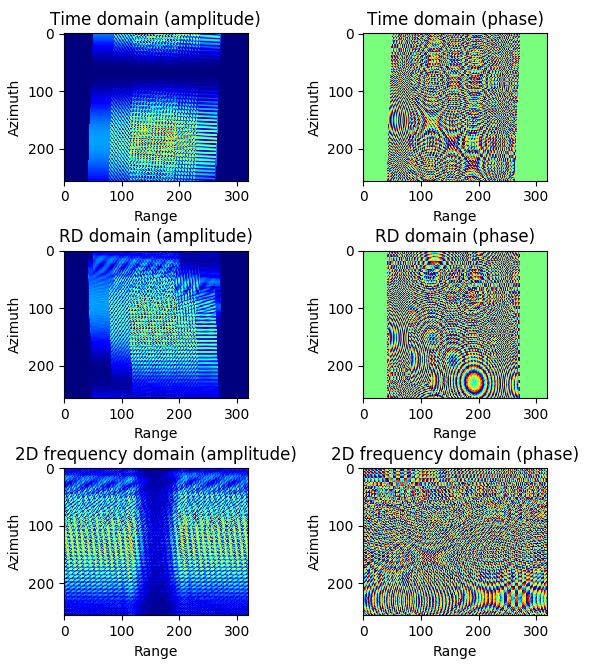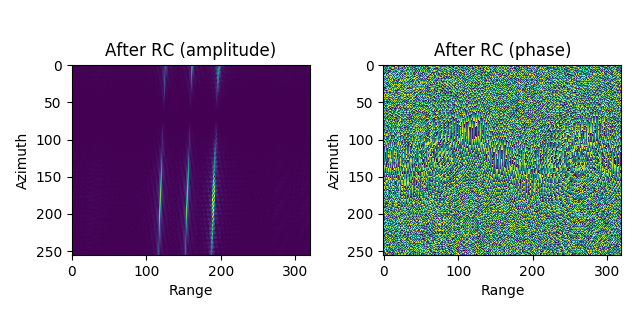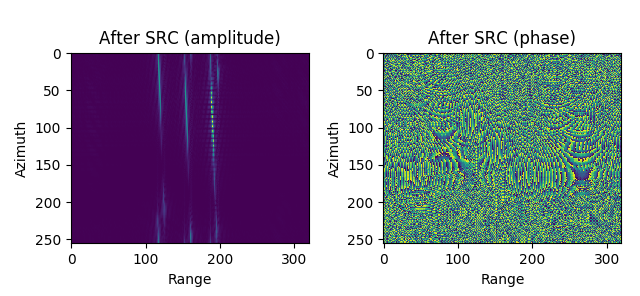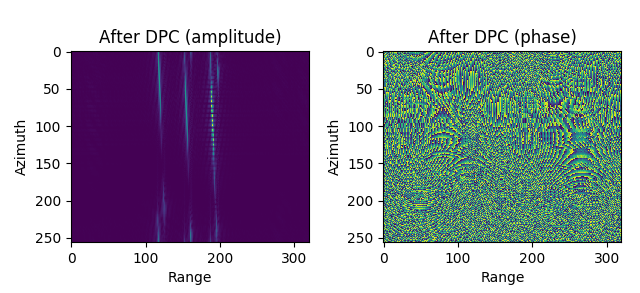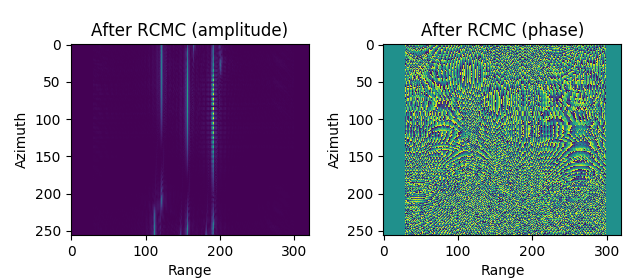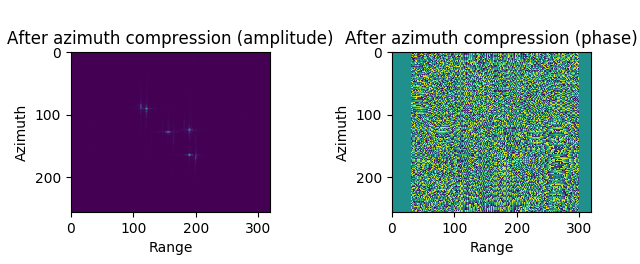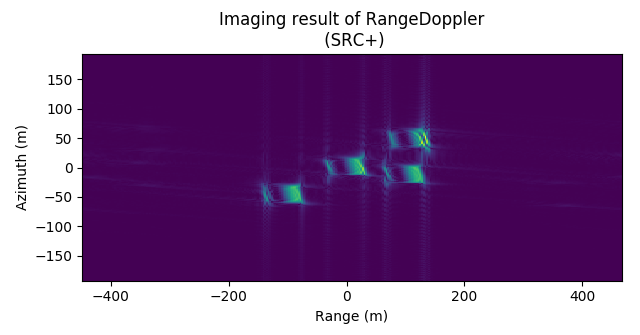RDA算法最终成像结果 (不含距离徙动校正)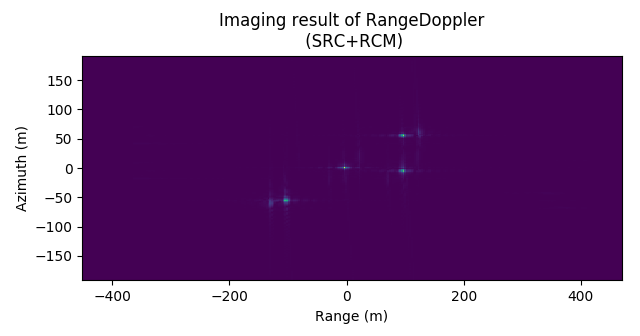RDA算法最终成像结果 (含距离徙动校正)

### 真实数据实验¶

#### 实验结果¶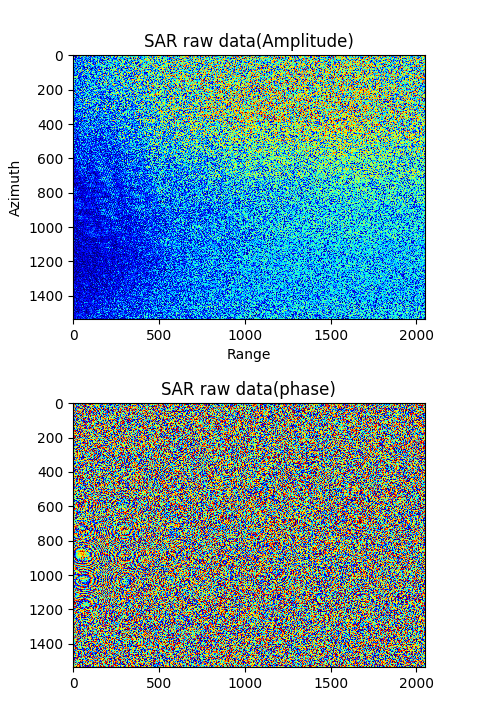SAR raw data amplitude and phase of Stanley Park.

RDA 成像结果 (以下结果均含多普勒相位补偿操作)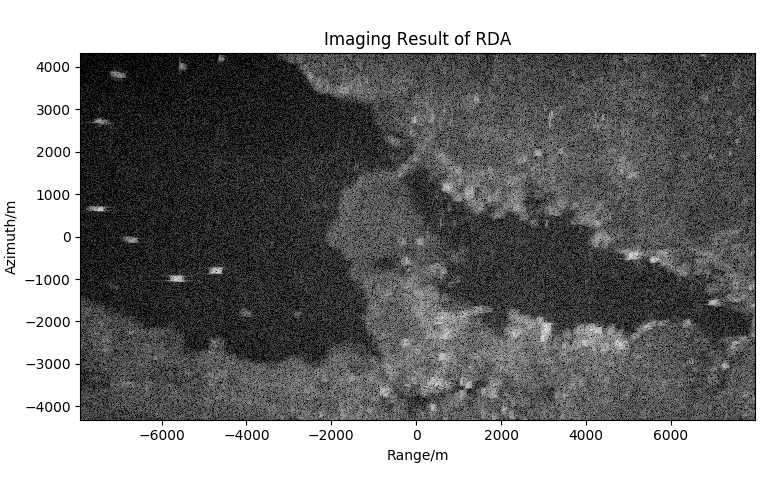Imaging result of RDA (without SRC, without RCMC)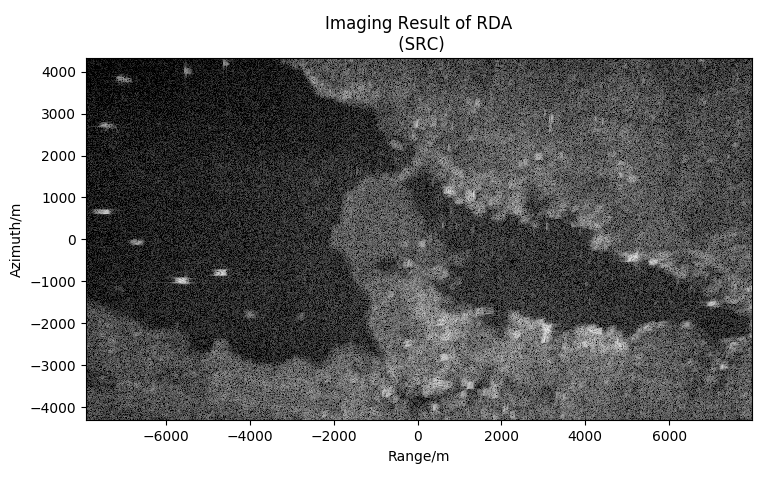Imaging result of RDA (with SRC, without RCMC)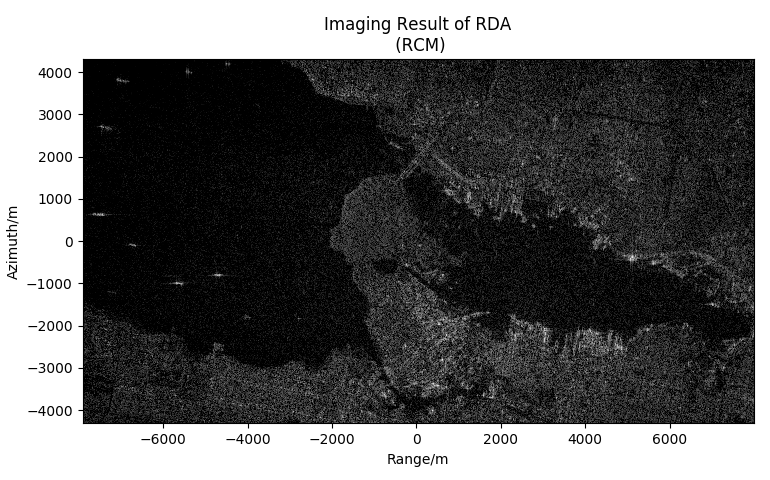Imaging result of RDA (without SRC, with RCMC(sinc 32))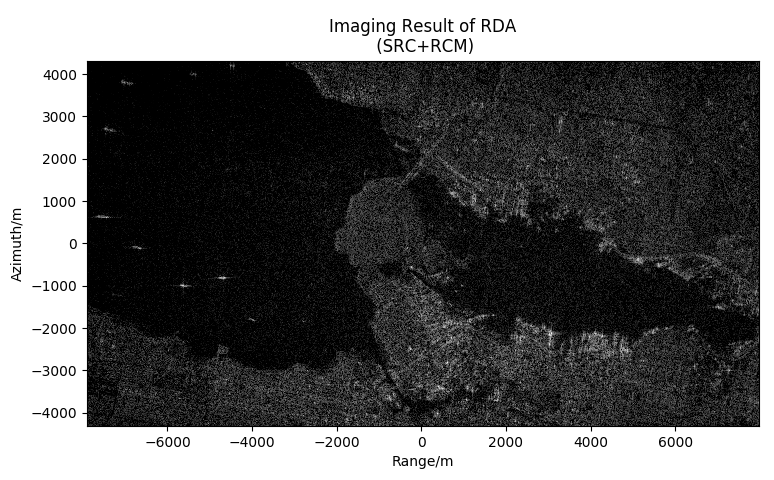Imaging result of RDA (with SRC, with RCMC(sinc 32))